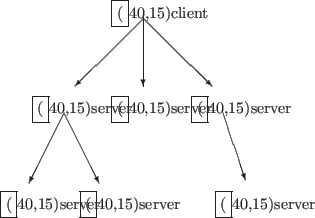### Nesting of client-server communication

Under OpenXM-RFC 100 an OpenXM server can be a client of other servers. Figure 9.2.3 illustrates a tree-like structure of an OpenXM client-server communication.Such a computational model is useful for parallel implementation of algorithms whose task can be divided into subtasks recursively. A typical example is quicksort, where an array to be sorted is partitioned into two sub-arrays and the algorithm is applied to each sub-array. In each level of recursion, two subtasks are generated and one can ask other OpenXM servers to execute them. Though it makes little contribution to the efficiency in the case of quicksort, we present an Asir program of this distributed quicksort to demonstrate that OpenXM gives an easy way to test this algorithm. In the program, a predefined constant LevelMax determines whether new servers are launched or whole subtasks are done on the server.

#define LevelMax 2
extern Proc1, Proc2;
Proc1 = -1$Proc2 = -1$

/* sort [A[P],...,A[Q]] by quicksort */
def quickSort(A,P,Q,Level) {
if (Q-P < 1) return A;
Mp = idiv(P+Q,2); M = A[Mp]; B = P; E = Q;
while (1) {
while (A[B] < M) B++;
while (A[E] > M && B <= E) E--;
if (B >= E) break;
else { T = A[B]; A[B] = A[E]; A[E] = T; E--; }
}
if (E < P) E = P;
if (Level < LevelMax) {
/* launch new servers if necessary */
if (Proc1 == -1) Proc1 = ox_launch(0);
if (Proc2 == -1) Proc2 = ox_launch(0);
/* send the requests to the servers */
ox_rpc(Proc1,"quickSort",A,P,E,Level+1);
ox_rpc(Proc2,"quickSort",A,E+1,Q,Level+1);
if (E-P < Q-E) {
A1 = ox_pop_local(Proc1);
A2 = ox_pop_local(Proc2);
}else{
A2 = ox_pop_local(Proc2);
A1 = ox_pop_local(Proc1);
}
for (I=P; I<=E; I++) A[I] = A1[I];
for (I=E+1; I<=Q; I++) A[I] = A2[I];
return(A);
}else{
/* everything is done on this server */
quickSort(A,P,E,Level+1);
quickSort(A,E+1,Q,Level+1);
return(A);
}
}


Another example is a parallelization of the Cantor-Zassenhaus algorithm for polynomial factorization over finite fields. It is a recursive algorithm similar to quicksort. At each level of the recursion, a given polynomial can be divided into two non-trivial factors with some probability by using a randomly generated polynomial as a separator. In the following program, one of the two factors generated on a server is sent to another server and the other factor is factorized on the server itself.

/* factorization of F */
/* E = degree of irreducible factors in F */
def c_z(F,E,Level)
{
V = var(F); N = deg(F,V);
if ( N == E ) return [F];
M = field_order_ff(); K = idiv(N,E); L = [F];
while ( 1 ) {
/* gererate a random polynomial */
W = monic_randpoly_ff(2*E,V);
/* compute a power of the random polynomial */
T = generic_pwrmod_ff(W,F,idiv(M^E-1,2));
if ( !(W = T-1) ) continue;
/* G = GCD(F,W^((M^E-1)/2)) mod F) */
G = ugcd(F,W);
if ( deg(G,V) && deg(G,V) < N ) {
/* G is a non-trivial factor of F */
if ( Level >= LevelMax ) {
/* everything is done on this server */
L1 = c_z(G,E,Level+1);
L2 = c_z(sdiv(F,G),E,Level+1);
} else {
/* launch a server if necessary */
if ( Proc1 < 0 ) Proc1 = ox_launch();
/* send a request with Level = Level+1 */
/* ox_c_z is a wrapper of c_z on the server */
ox_cmo_rpc(Proc1,"ox_c_z",lmptop(G),E,
setmod_ff(),Level+1);
/* the rest is done on this server */
L2 = c_z(sdiv(F,G),E,Level+1);
L1 = map(simp_ff,ox_pop_cmo(Proc1));
}
return append(L1,L2);
}
}
}


Nobuki Takayama 2017-03-30